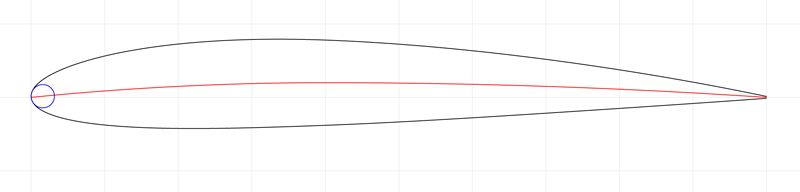Nukem's Possible Manifestation

# npm

## naca-four-digit-airfoil

1.0.4 • Public • Published

# naca-four-digit-airfoil

The equations for a NACA four-digit series airfoil

## Introduction

NACA airfoils map a four digit code to an airfoil shape. For example, a '2412' airfoil is parsed to mean:

• The leading '2' indicates maximum camber is 2% of chord length
• The '4' indicates maximum camber is at 40% of the chord length
• The '12' indicates maximum thickness is 12% of the chord lengthLive examples (change the query string to create your own):

## Example

The code below calculates the x- and y-coordinates of the upper and lower surfaces, respectively, at 50% of the chord length.

## API

### Based on NACA Code

#### `require('naca-four-digit-airfoil')(nacaAirfoilCode[, chordLength = 1])`

Parses the airfoil code and returns an object with functions that evaluate the shape of the airfoil for a unit chord length. Arguments are:

• `nacaAirfoilCode`: A four-digit string containing the NACA airfoil code. The first digit is the amount of camber in units of 1% of the chord length. The second digit is the location of maximum camber measured from the leading edge in units of 10% of the chord length. The final two digits are the thickness of the airfoil in units of 1% of the chord length.
• `chordLength` (optional, default = 1): The chord length of the airfoil.

The object returned contains the following functions:

• `thickness: function(x)`: The half-thickness of the airfoil above or below the camber line at position x.
• `camberLine: function(x)`: The height of the camber line above the centerline at position `x`.
• `camberLineSlope: function(x)`: The slope of the camber line at position `x`.
• `leadingEdgeRadius: function()`: The radius of curvature of the leading edge.
• `evaluate: function(x)`: The coordinates of the surface at position `x`. Returned in a four-element array containing coordinates `[xUpper, yUpper, xLower, yLower]`. The coordinates are calculated perpendicular to the line of camber. So that the x-coordinate of the surface at chord position `x` is slightly perturbed.
• `xUpper: function(x)`: The x-coordinate of the upper surface of the airfoil.
• `yUpper: function(x)`: The y-coordinate of the upper surface of the airfoil.
• `xLower: function(x)`: The x-coordinate of the lower surface of the airfoil.
• `yLower: function(x)`: The y-coordinate of the lower surface of the airfoil.

### As Plain Functions

The functions are also available as unadorned functions accessible on the plain module. Using the variables defined above, they are:

• `parse`: `function(code)`: Parse the four-character `String` airfoil code, returning `m` (the amount of camber as a fraction of chord length), `p` (the location of maximum camber as a fraction of chord length), and `t` (thickness as a fraction of chord length).
• `thickness`: `function(x, c, t)`
• `camberLine`: `function(x, c, m, p)`
• `camberLineSlope`: `function(x, c, m, p)`
• `leadingEdgeRadius`: `function(t, c)`
• `xLower`: `function(x, c, m, p, t)`
• `xUpper`: `function(x, c, m, p, t)`
• `yLower`: `function(x, c, m, p, t)`
• `yUpper`: `function(x, c, m, p, t)`
• `evaluate`: `function(x, c, m, p, t)`

## Keywords

### Install

`npm i naca-four-digit-airfoil`

### Repository

github.com/rreusser/naca-four-digit-airfoil